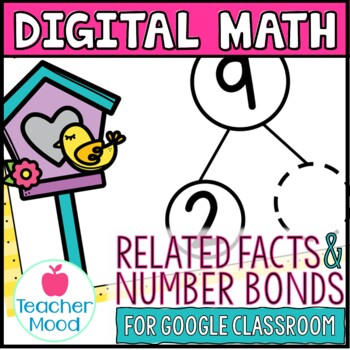# Number Bonds and Related Facts Digital Math Google Classroom Distance LearningGrade Levels
1st - 2nd
Subjects
Standards
Resource Type
Formats Included
• PDF
• Internet Activities
•Google Apps™
Pages
34 slidesIncludes Google Apps™
The Teacher-Author indicated this resource includes assets from Google Workspace (e.g. docs, slides, etc.).

### Description

This is a DIGITAL, Google Slides file perfect for distance learning. NO PREP OR PAPER NEEDED! The slide deck features 34 slides with number bond and related facts problems complete with moveable pieces and text boxes for students to input answers. This resource is perfect for first grade and below level second grade students and can easily be assigned in Google Classroom.

You may also like these resources for Google Classroom™️

✅✅✅✅✅✅✅✅✅✅✅✅✅✅✅✅✅✅✅

✅✅✅✅✅✅✅✅✅✅✅✅✅✅✅✅✅✅✅

You will get:

A pdf file that includes links to the digital, Google Slides resource and answer key. Please make sure that you're logged in to the Google account that you plan to use the resource with. Many school districts use Google Apps for Education. If so, please make sure you're logged in to your school account, and not your personal account.

You will need:

•Internet access

•a Google account

•a Google Classroom

Your students will need:

•Access to technology

•Internet access

•a Google account

You may also like-

Addition & Subtraction (RTI Progress Monitoring)

Part Part Whole Missing Addend Practice

Spoons Game {Math} Subtraction Facts - Differences within 20

Spoons Game {Math} Addition Facts - Sums to 20

Let's Get Connected!

Follow me on TPT!

Follow me on FACEBOOK!

Follow me on INSTAGRAM!

Follow me on PINTEREST!

Follow me on MY BLOG!

Total Pages
34 slides
Answer Key
Included
Teaching Duration
N/A
Report this Resource to TpT
Reported resources will be reviewed by our team. Report this resource to let us know if this resource violates TpT’s content guidelines.

### Standards

to see state-specific standards (only available in the US).
Fluently add and subtract within 20 using mental strategies. By end of Grade 2, know from memory all sums of two one-digit numbers.
Determine the unknown whole number in an addition or subtraction equation relating three whole numbers. For example, determine the unknown number that makes the equation true in each of the equations 8 + ? = 11, 5 = ▯ - 3, 6 + 6 = ▯.
Add and subtract within 20, demonstrating fluency for addition and subtraction within 10. Use strategies such as counting on; making ten (e.g., 8 + 6 = 8 + 2 + 4 = 10 + 4 = 14); decomposing a number leading to a ten (e.g., 13 - 4 = 13 - 3 - 1 = 10 - 1 = 9); using the relationship between addition and subtraction (e.g., knowing that 8 + 4 = 12, one knows 12 - 8 = 4); and creating equivalent but easier or known sums (e.g., adding 6 + 7 by creating the known equivalent 6 + 6 + 1 = 12 + 1 = 13).
Use addition and subtraction within 20 to solve word problems involving situations of adding to, taking from, putting together, taking apart, and comparing, with unknowns in all positions, e.g., by using objects, drawings, and equations with a symbol for the unknown number to represent the problem.

### Questions & Answers

Teachers Pay Teachers is an online marketplace where teachers buy and sell original educational materials.

More About Us